Learn to click the perfect selfie Learn to click the perfect selfie

# ICSE Class 10 Maths Mock Sample Question Paper 4 With Answers - Free PDF Download

ICSE Class 10 Maths Sample Question Paper – Mock 4 with Answers are given at BYJU’S that can be accessed in a pdf and online at any time. Students can download the solutions of sample papers for class 10 maths which are prepared by the subject experts with accurate answers and in an easily understandable way.

The best tips for cracking the ICSE maths class 10 exam include referring books that cover the syllabus, solving previous years board papers of ICSE and practising as many questions as possible. This will help the students to identify the right approach for solving any type of problem in the examination.

#### ICSE Class 10 Maths Mock Sample Paper 4 with Solutions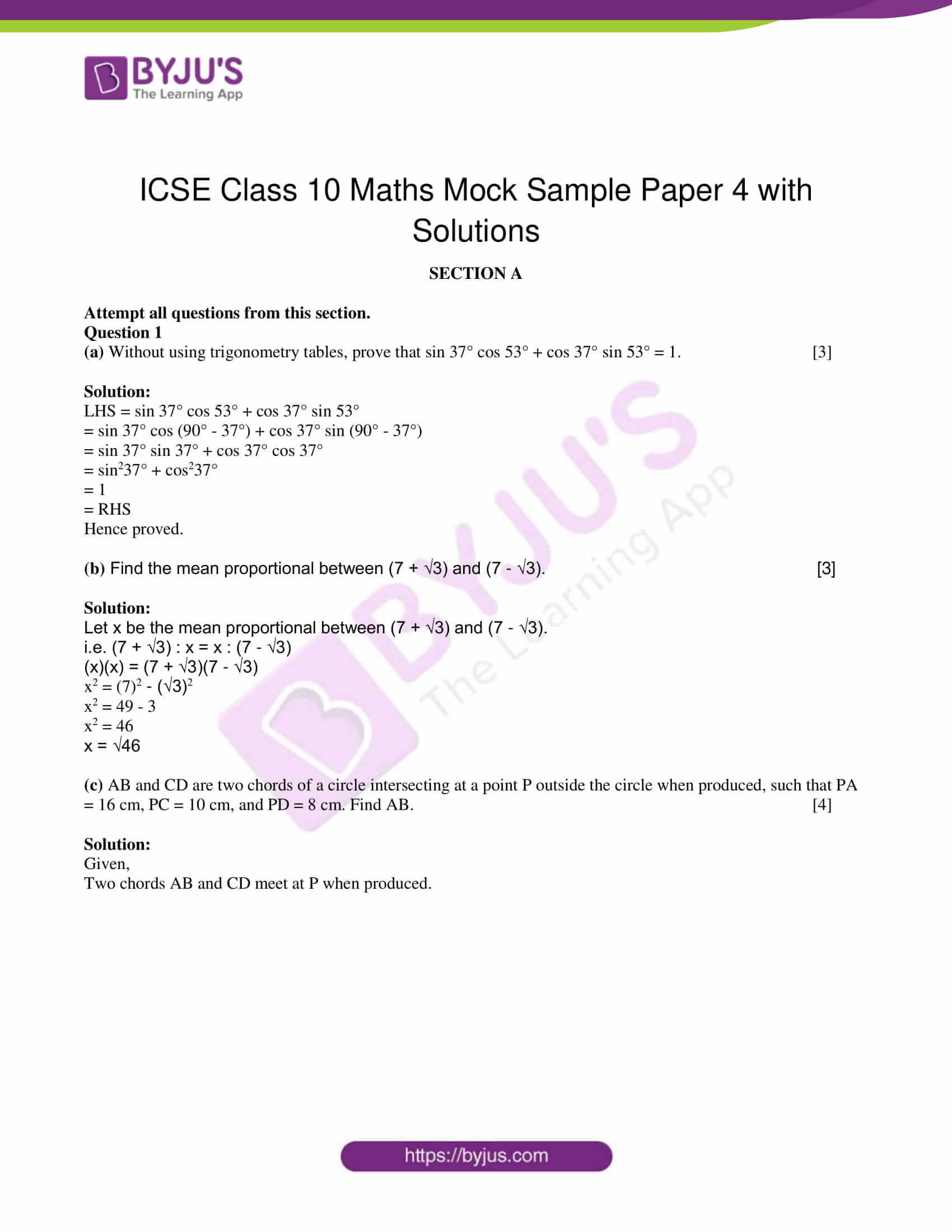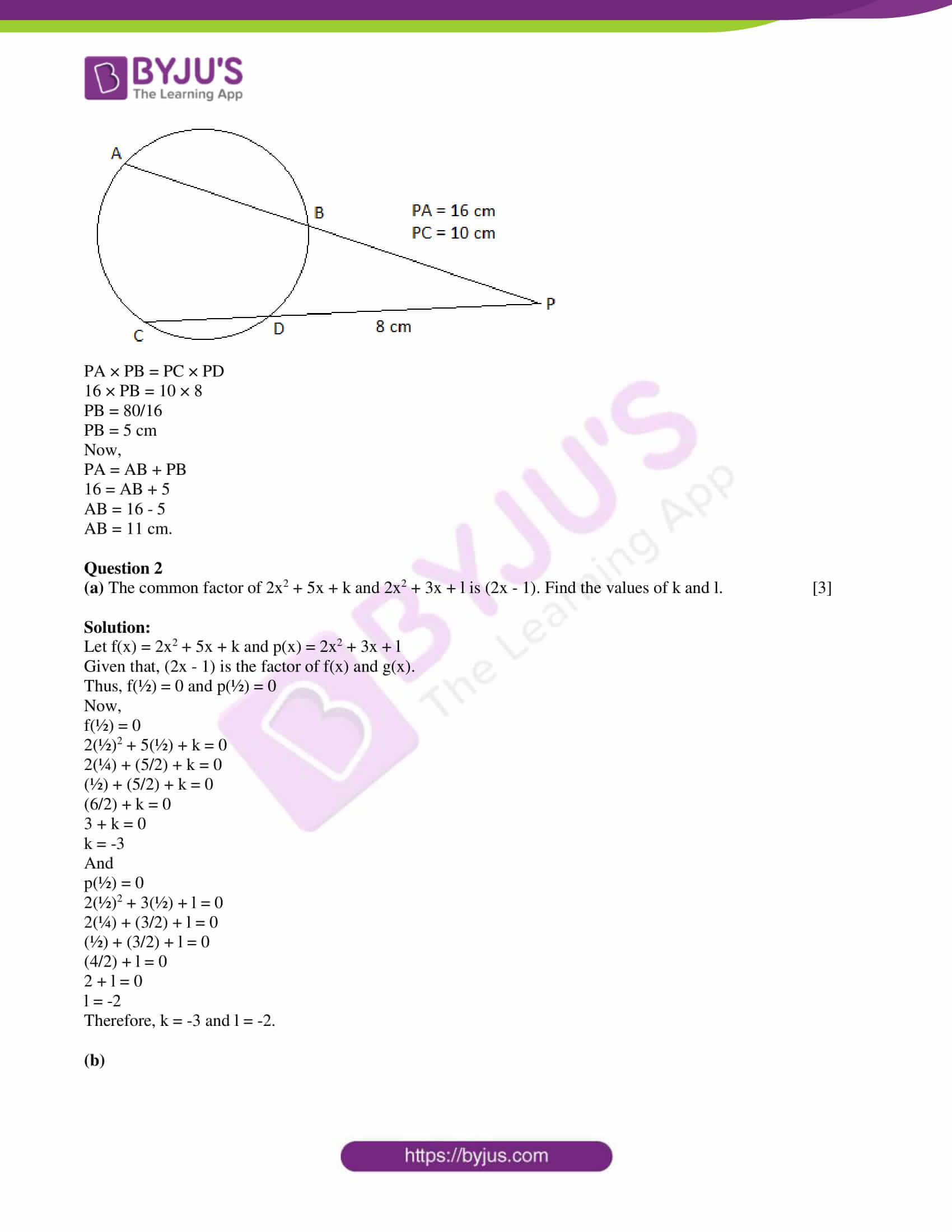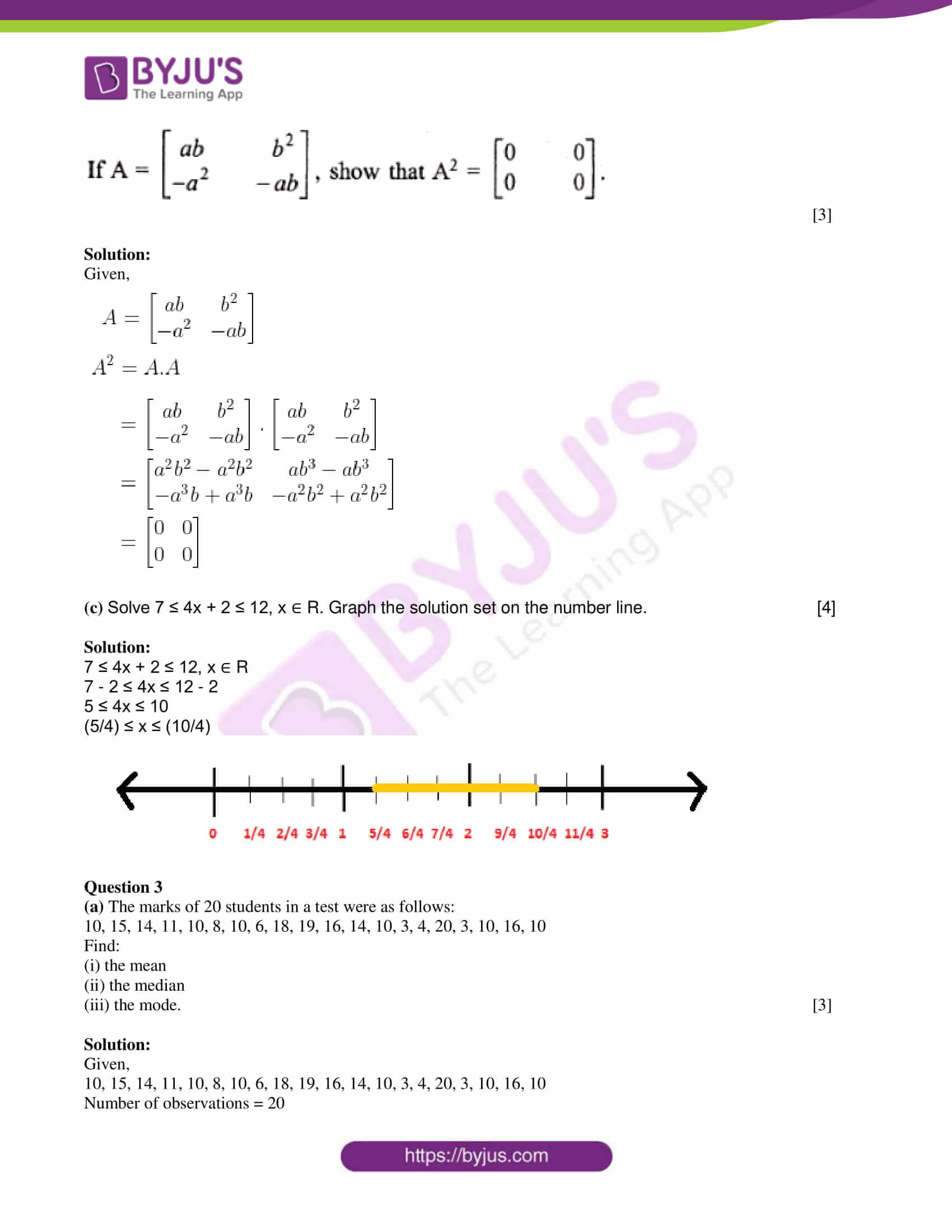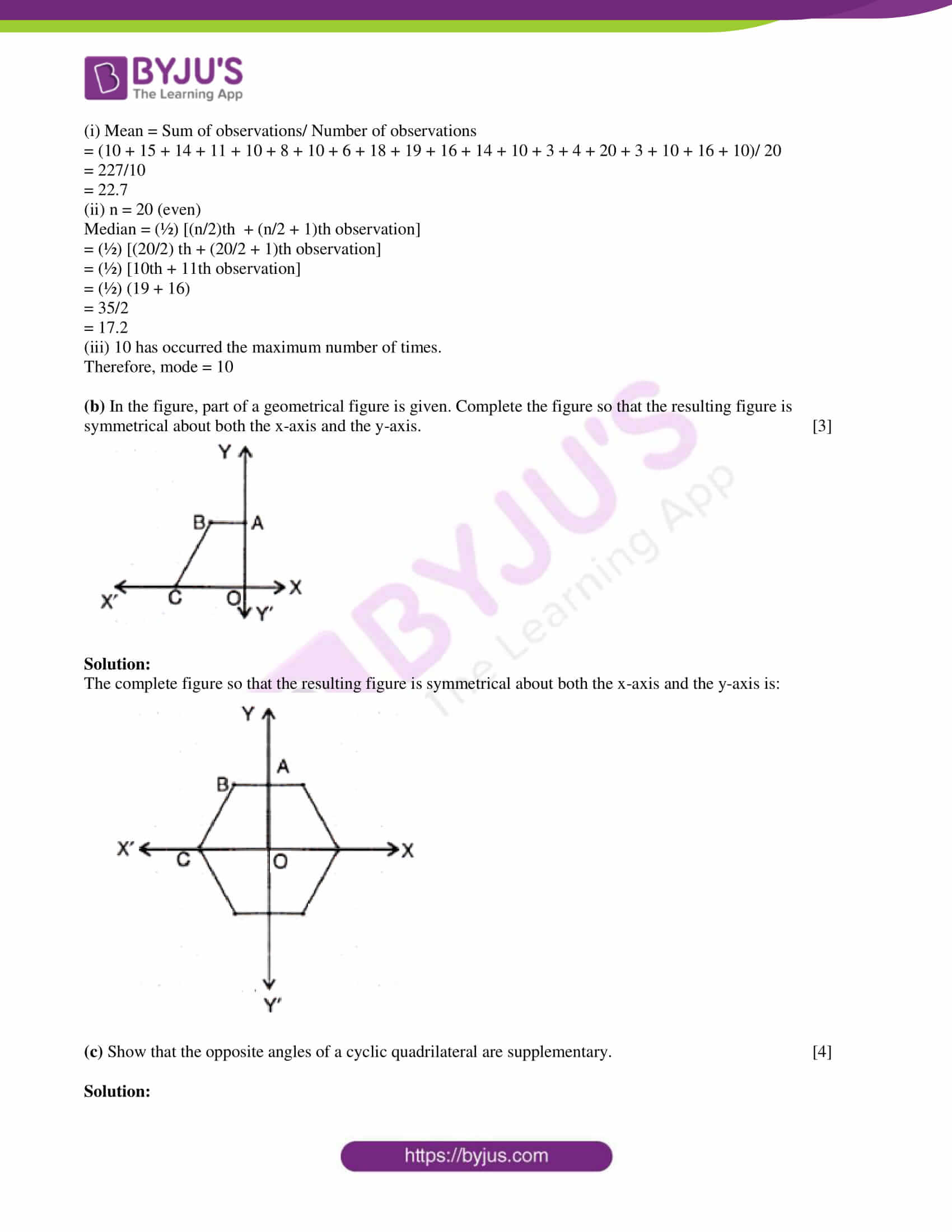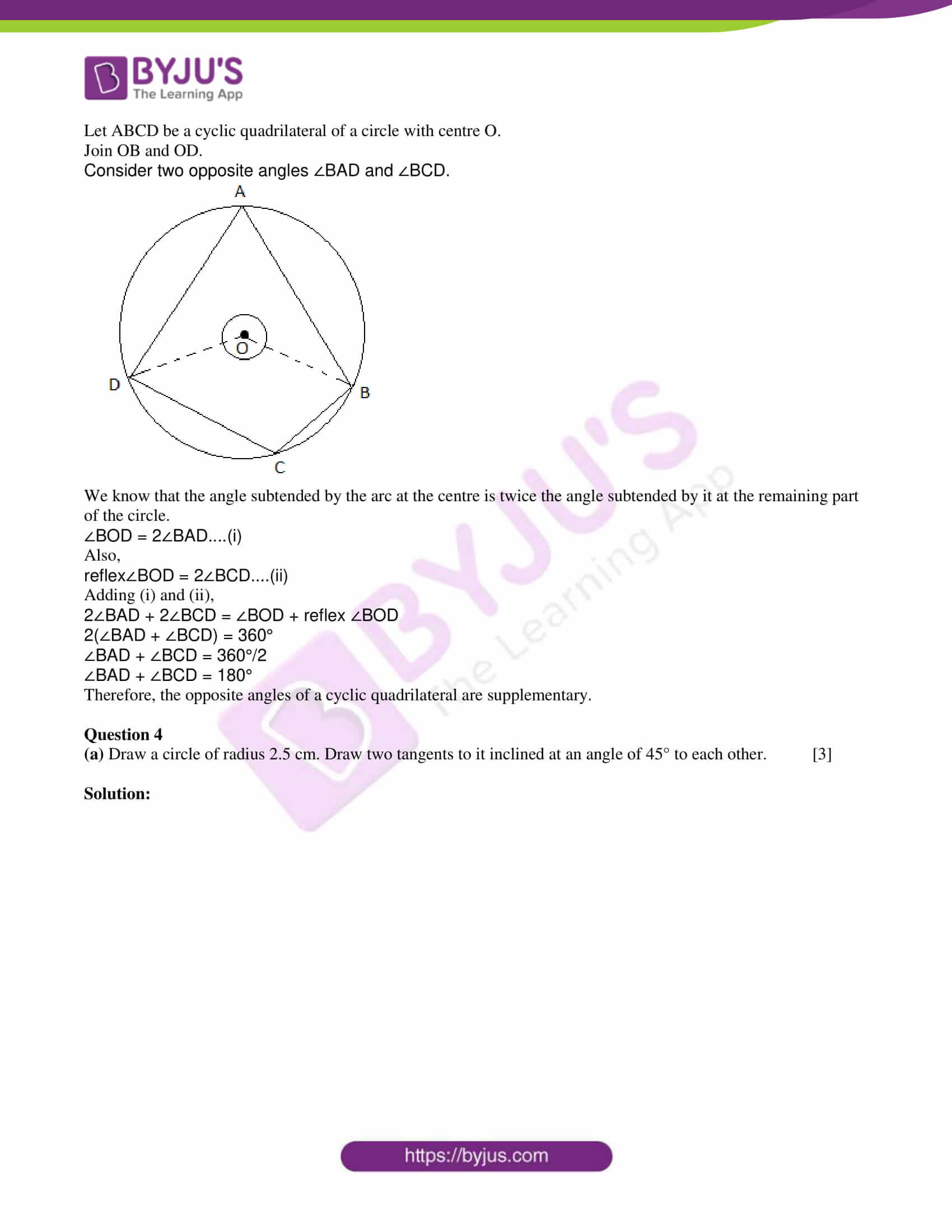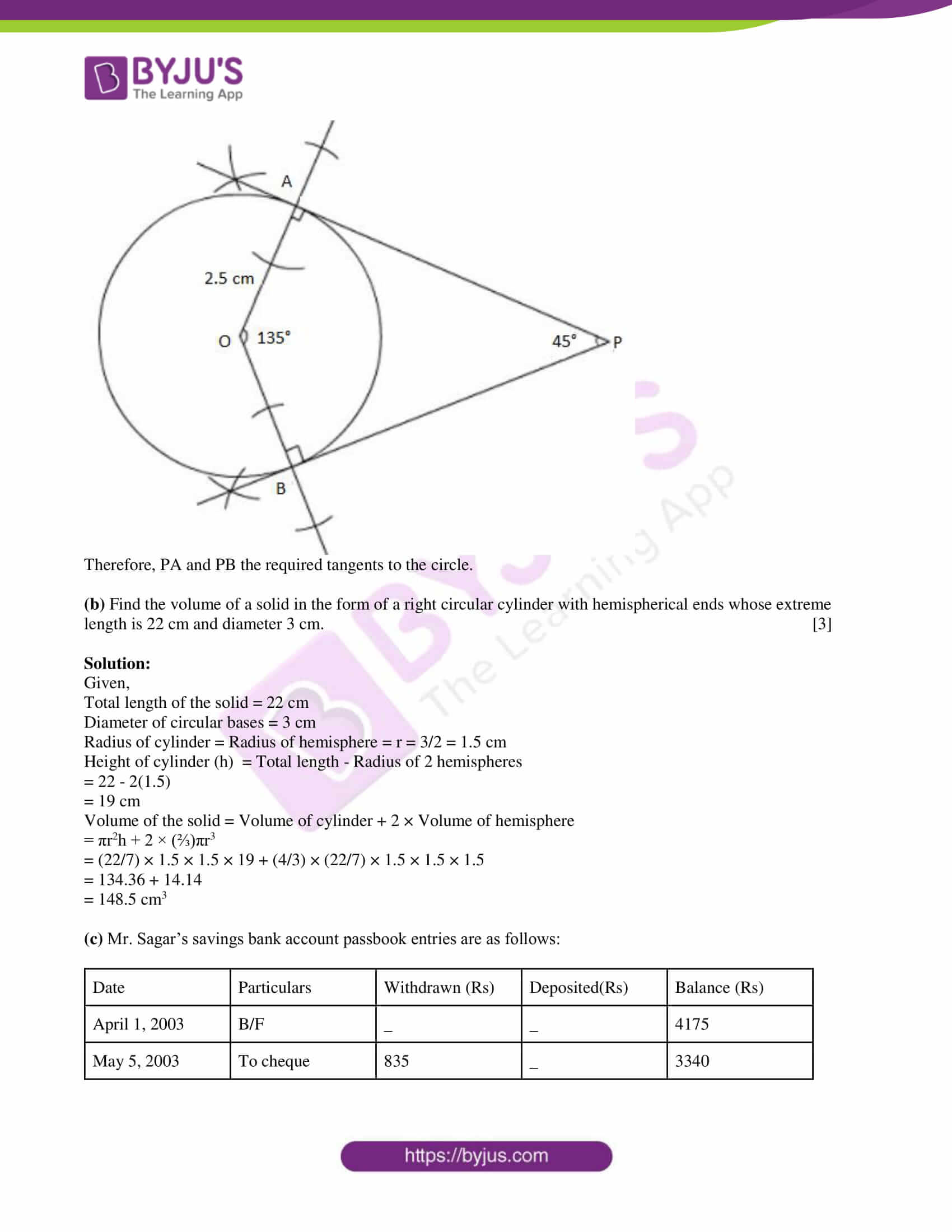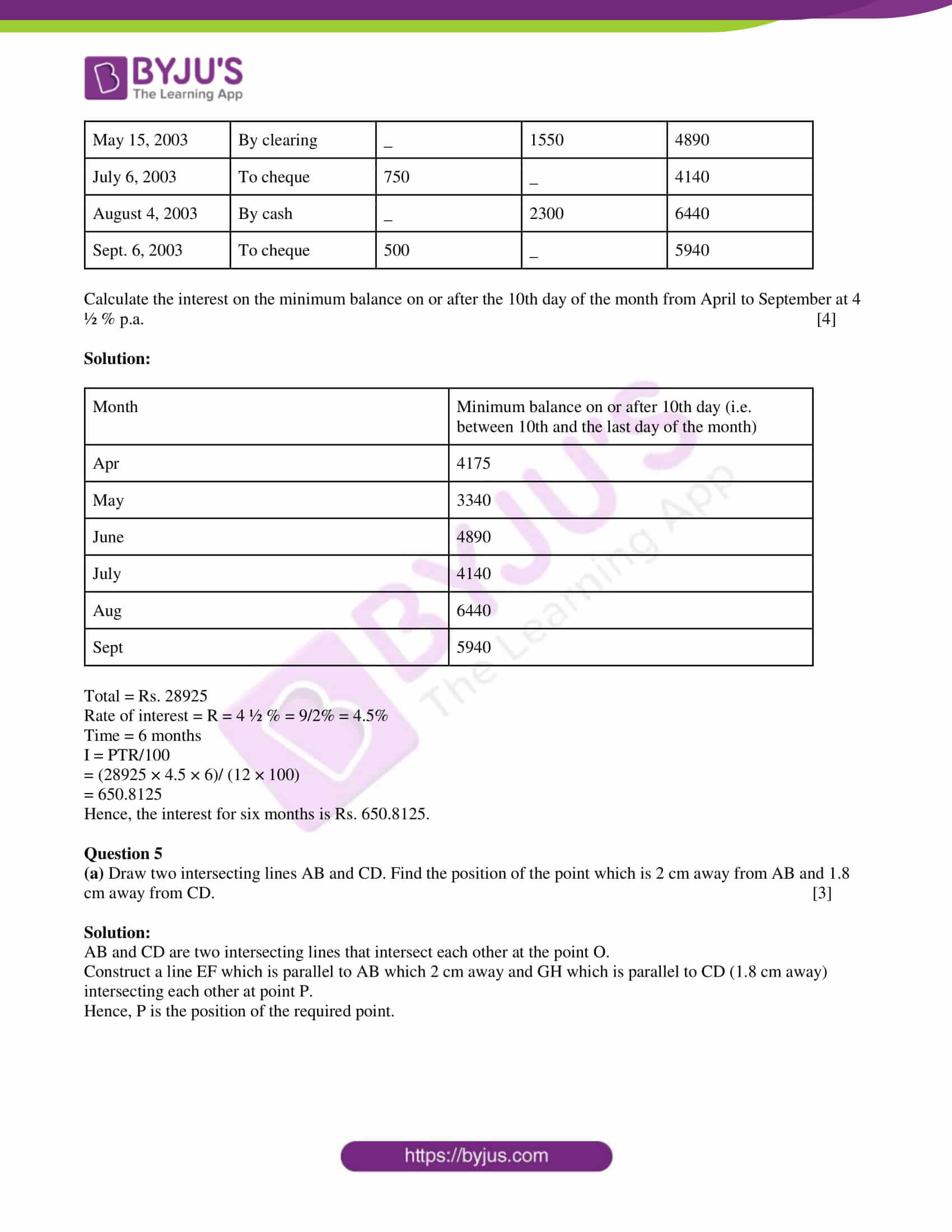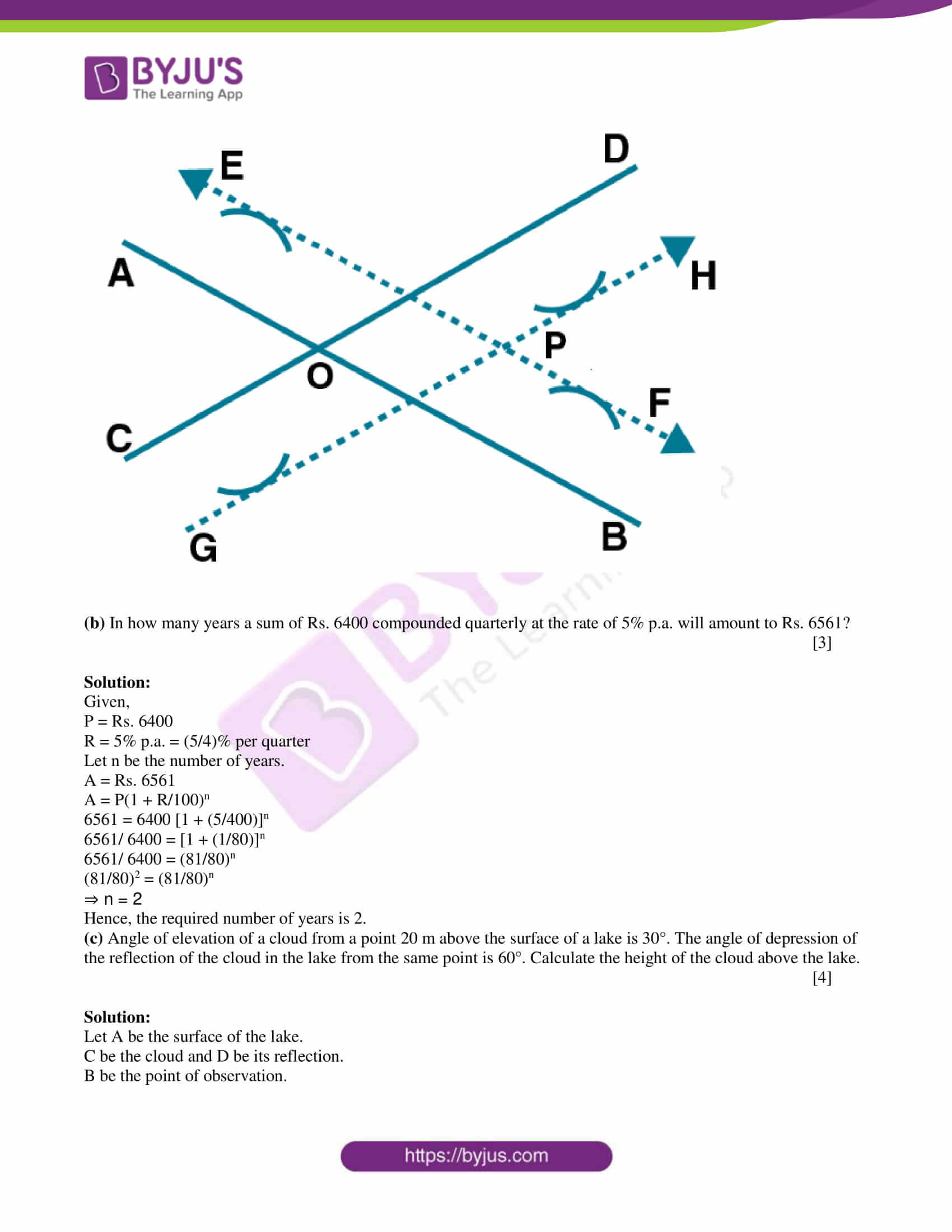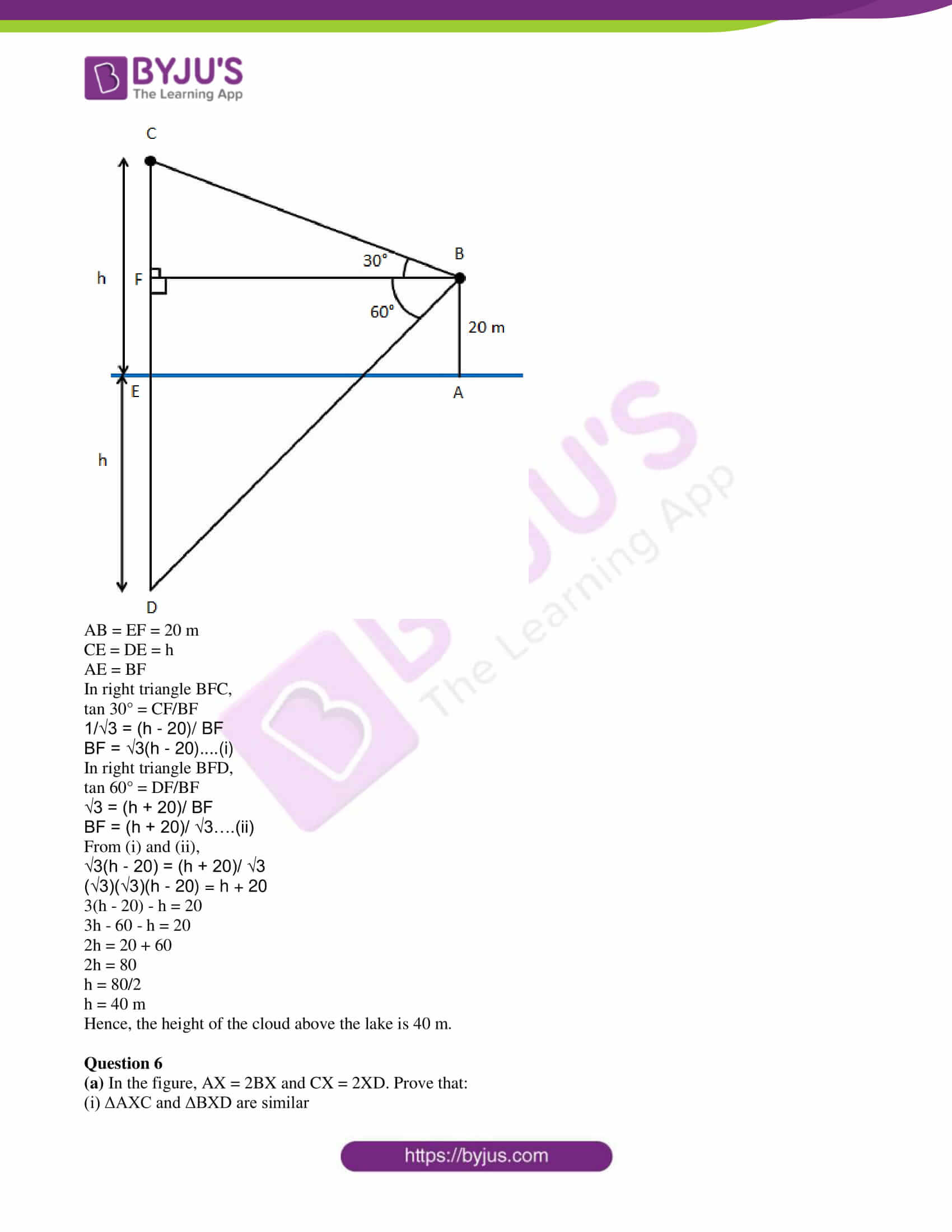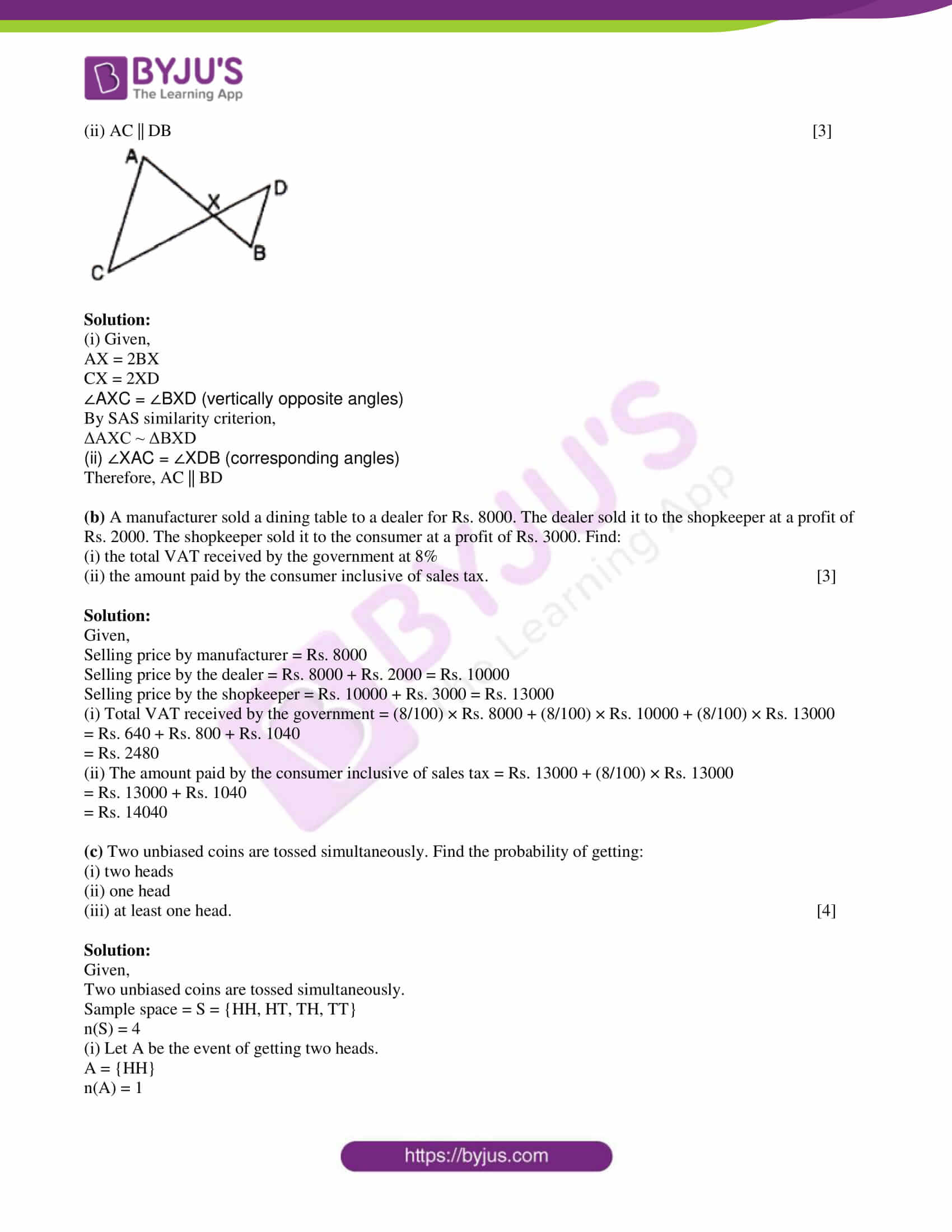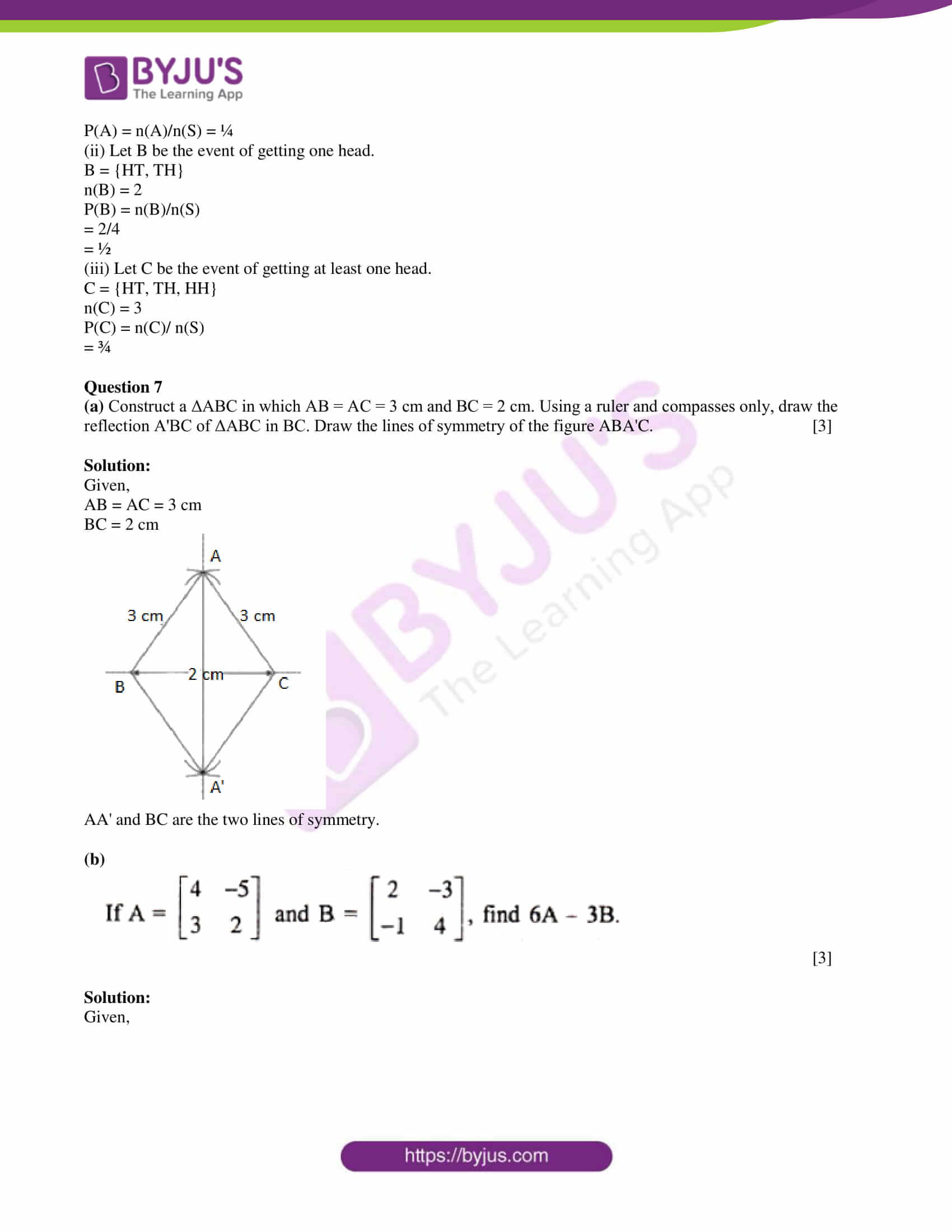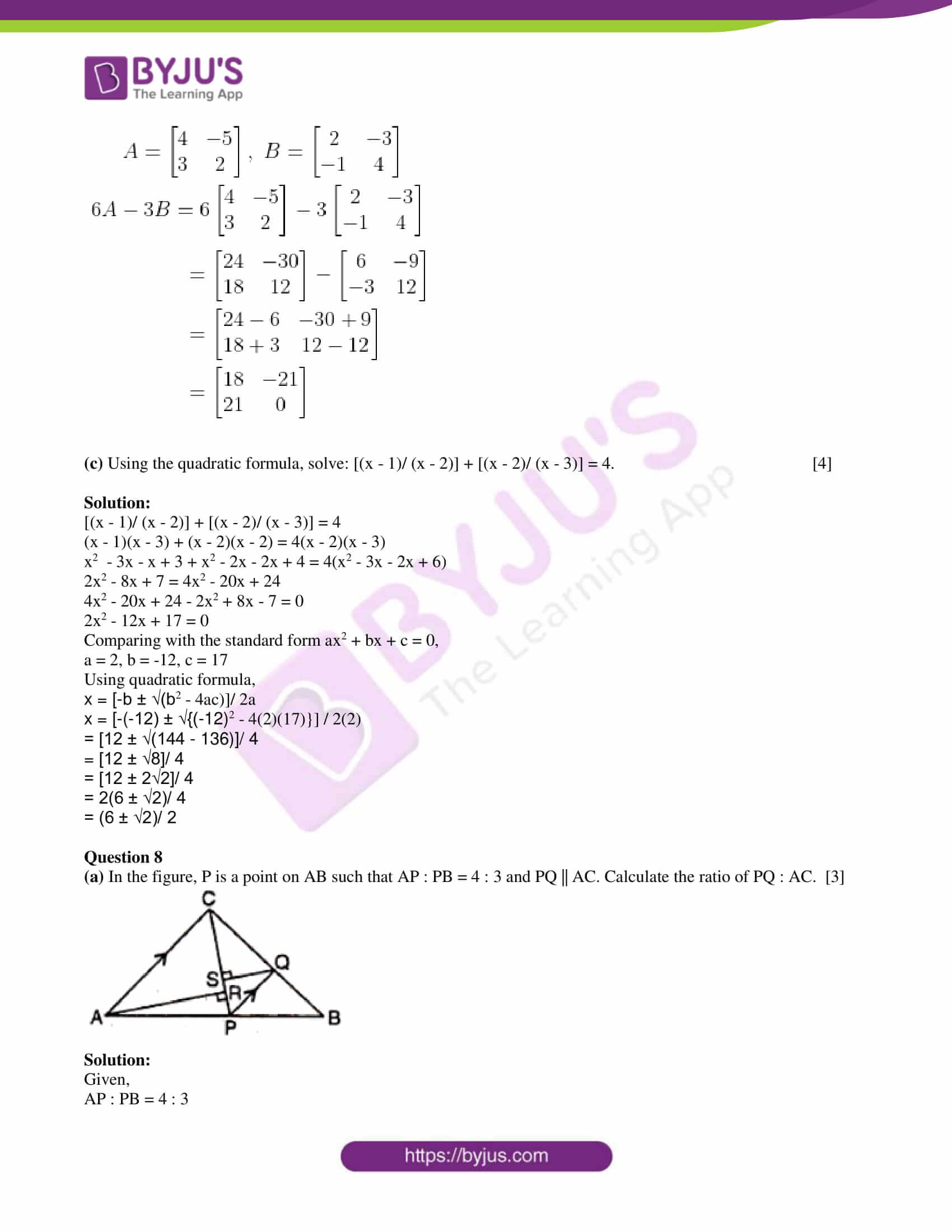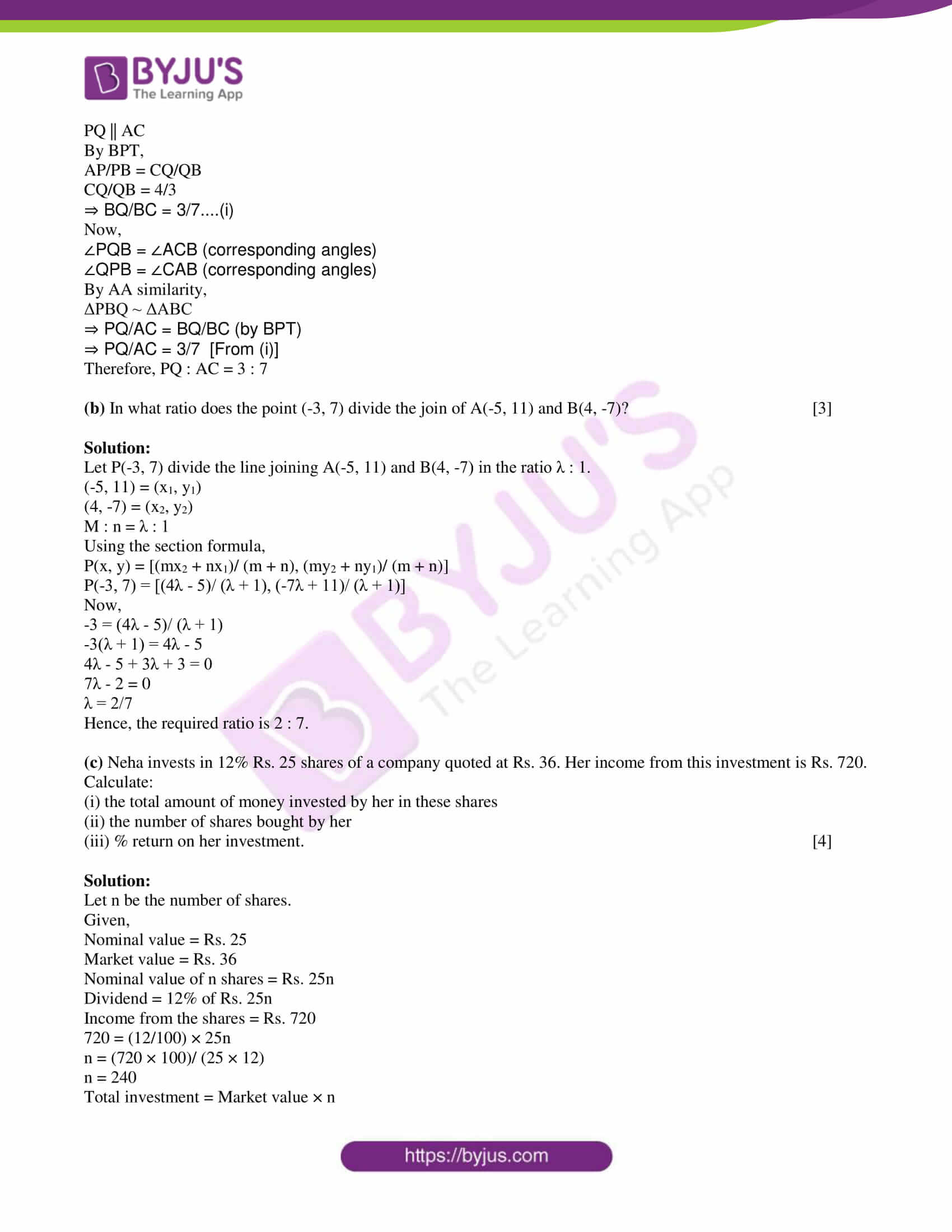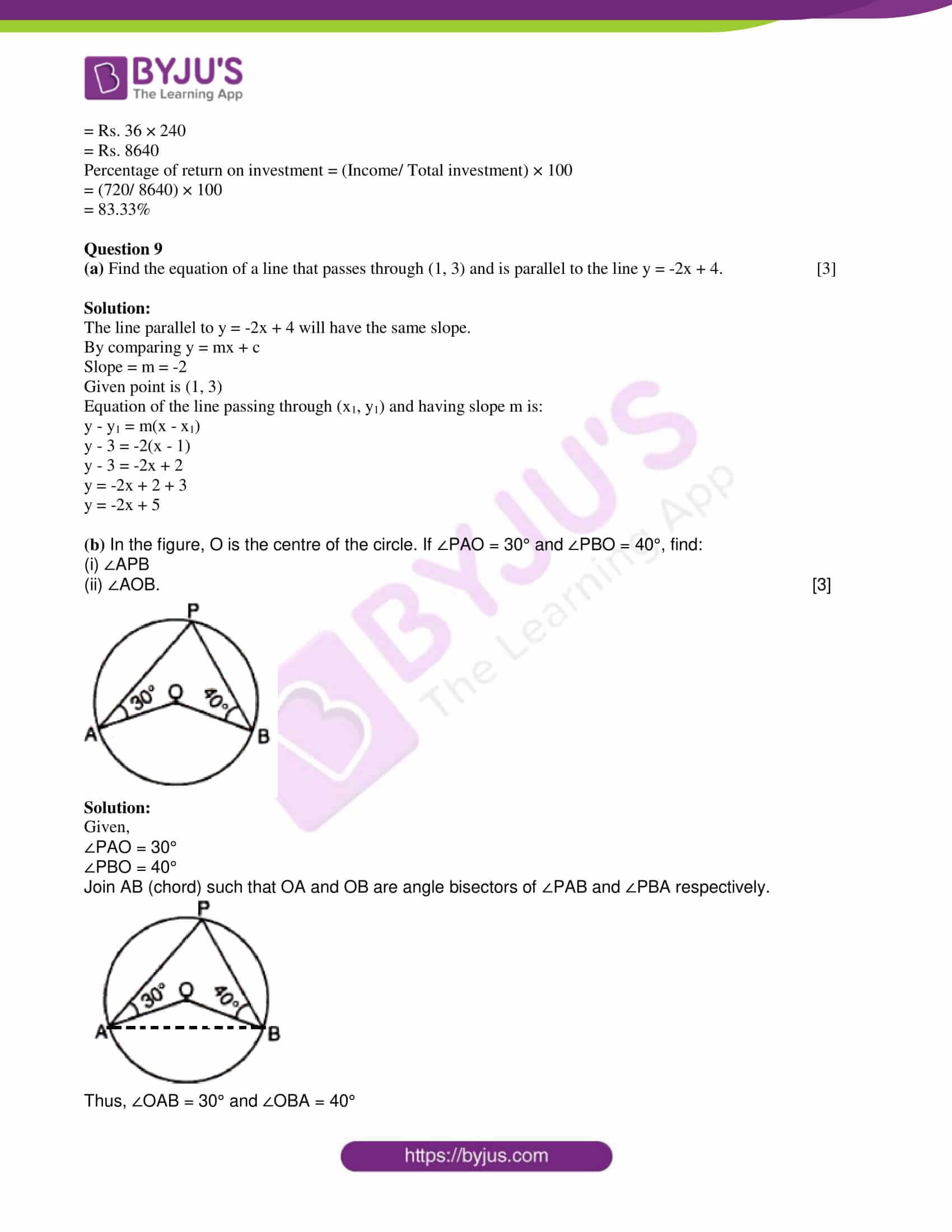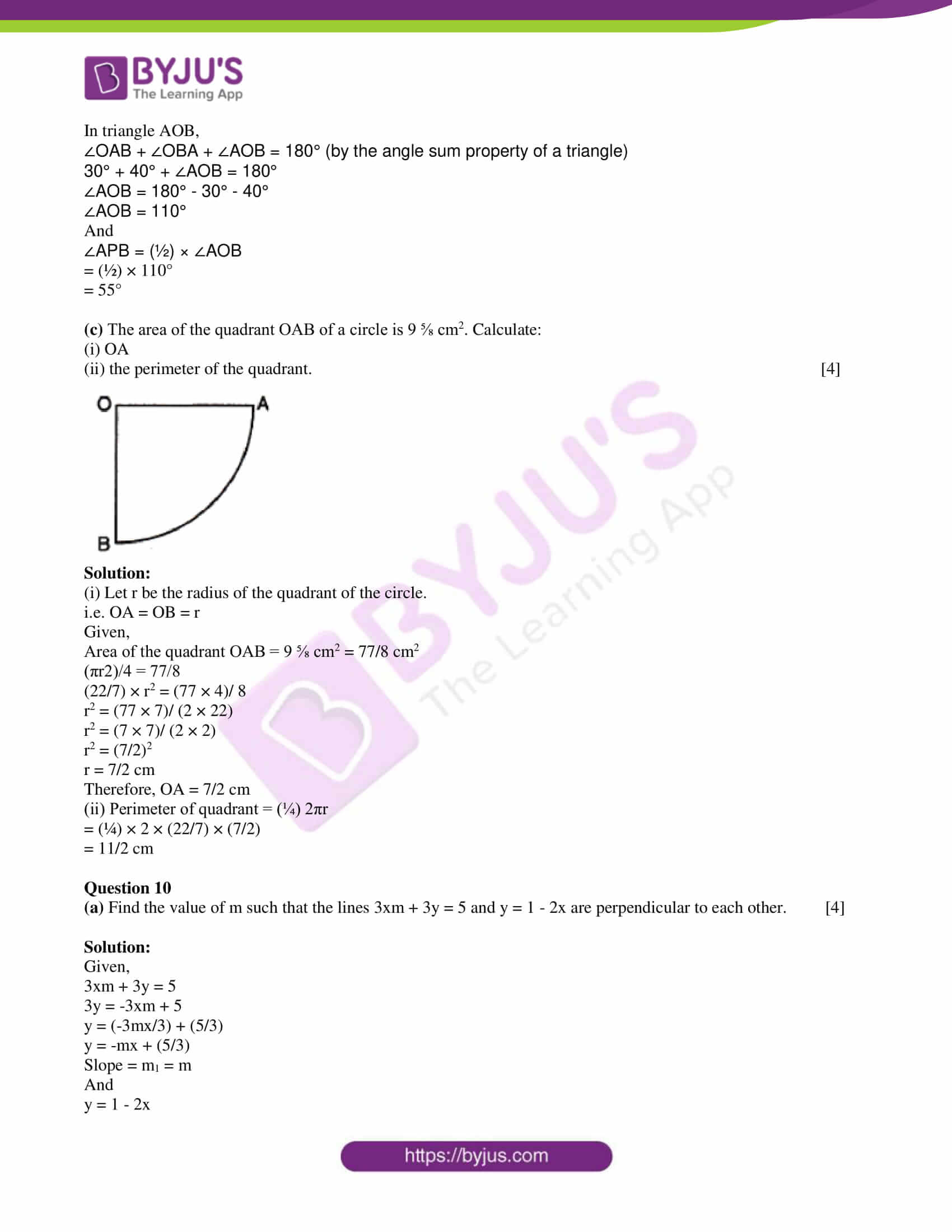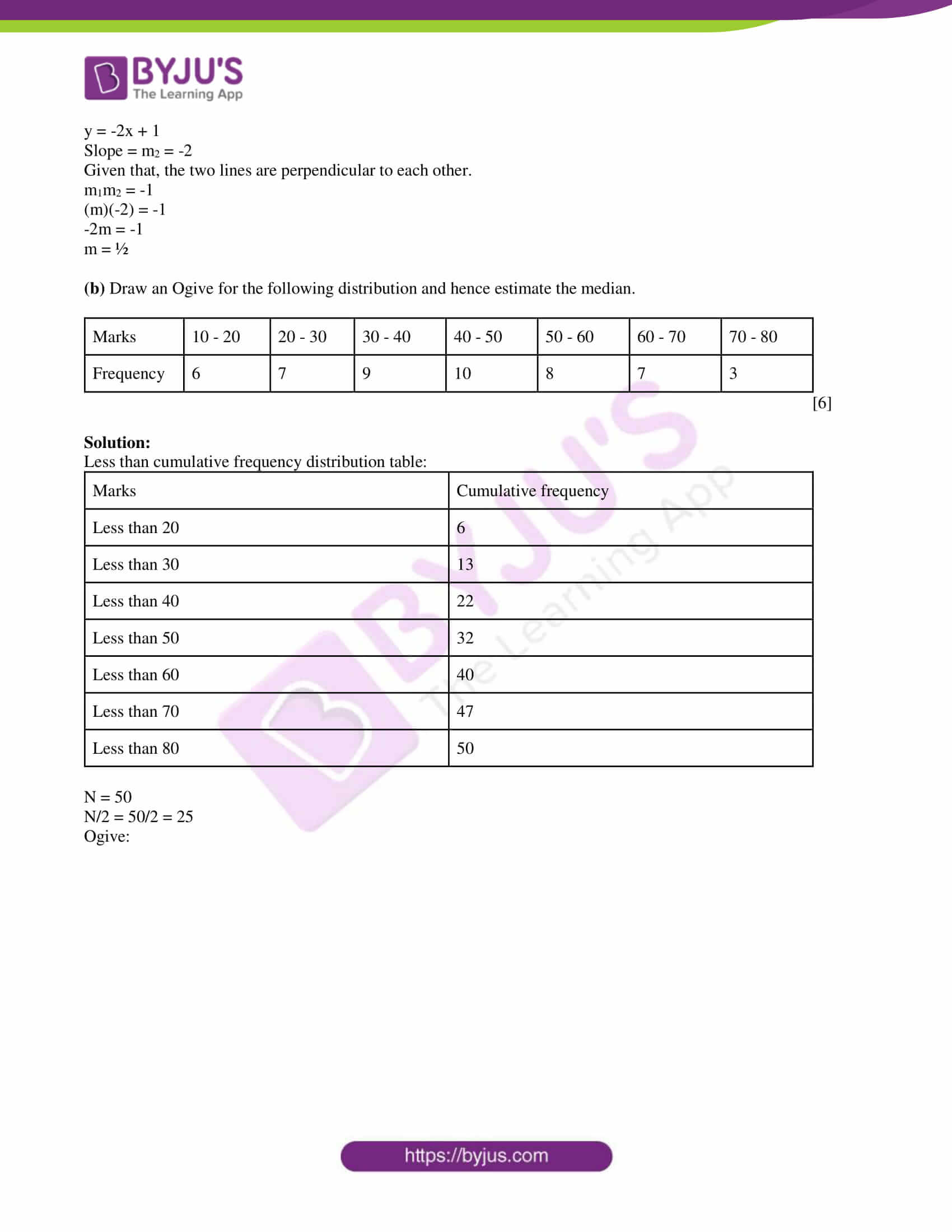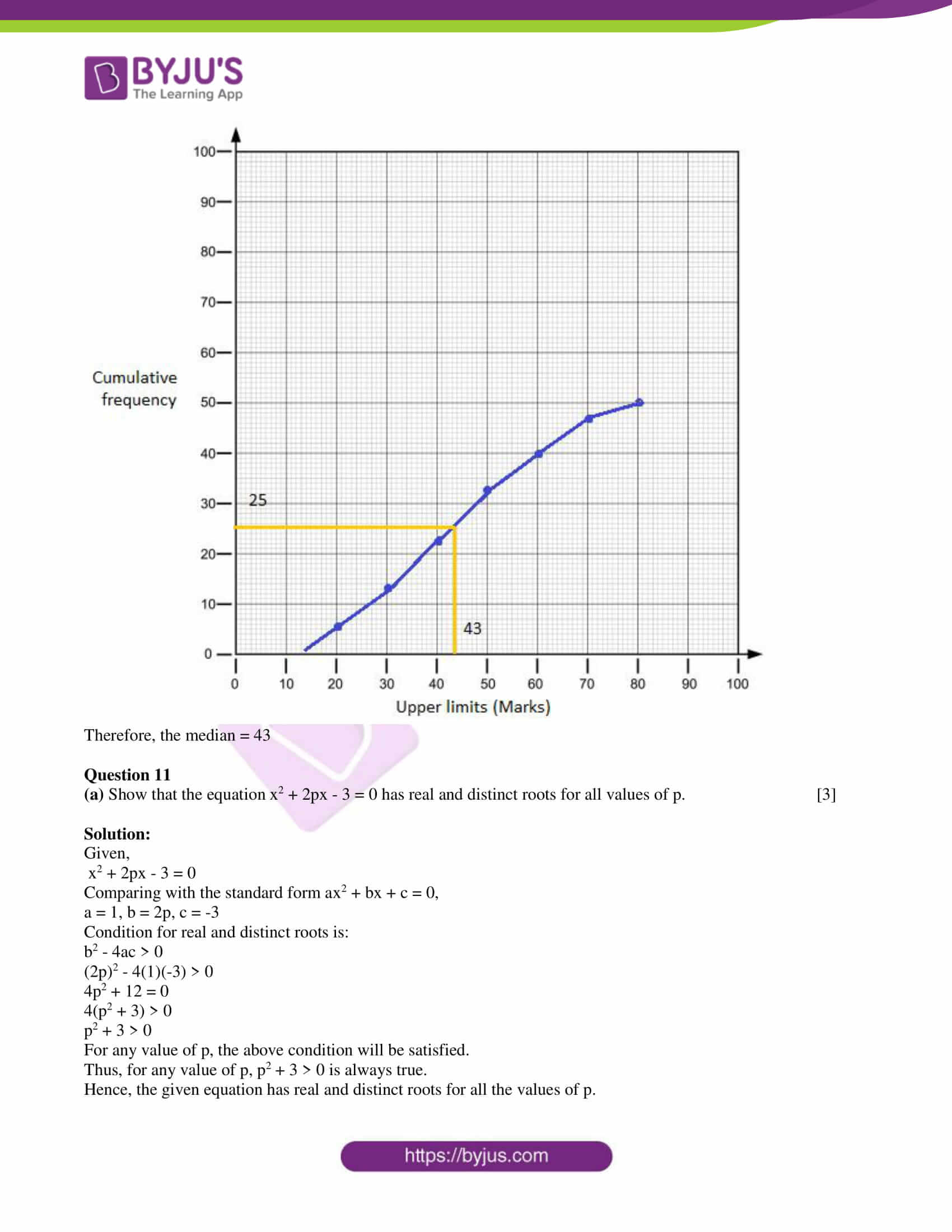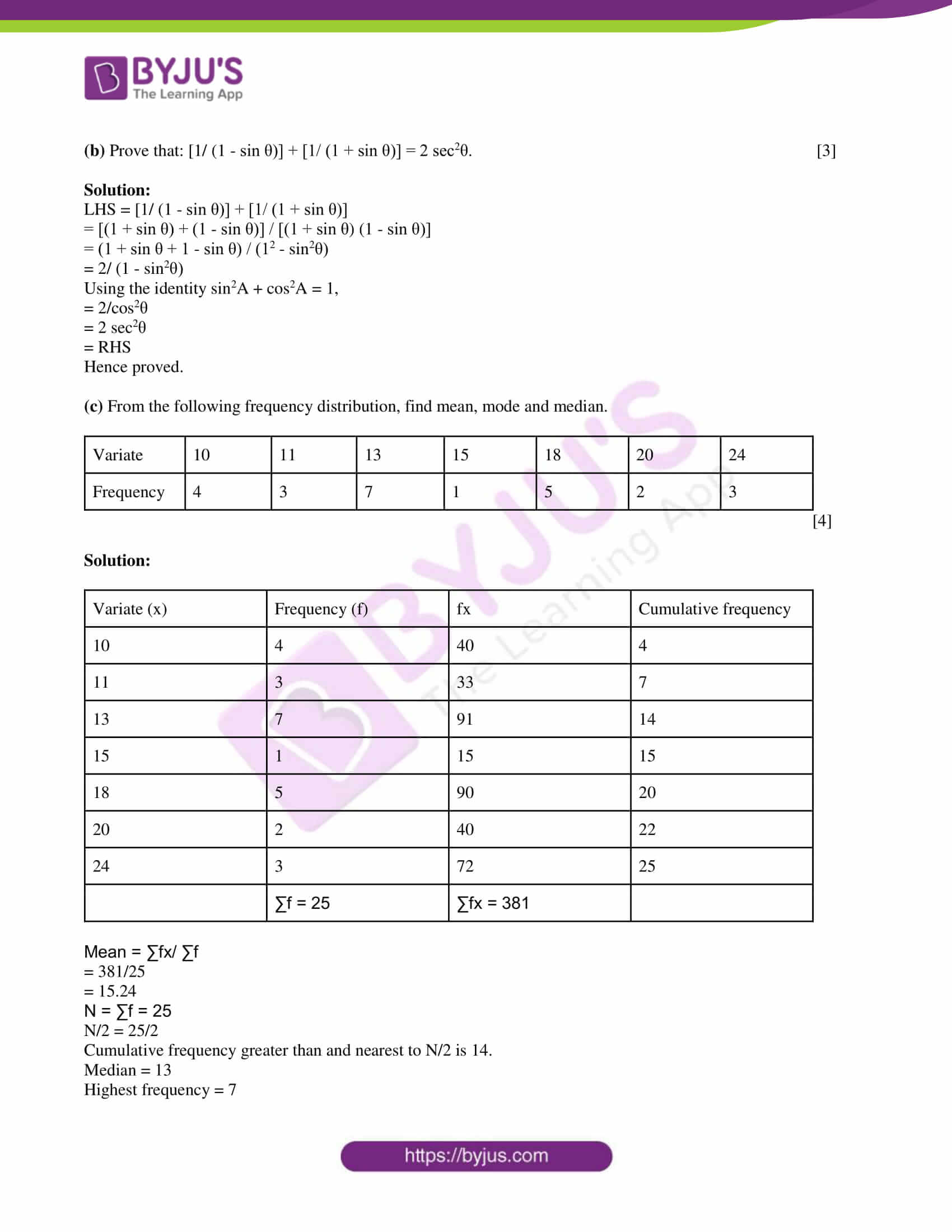SECTION A

Attempt all questions from this section.

Question 1

(a) Without using trigonometry tables, prove that sin 37° cos 53° + cos 37° sin 53° = 1. 

Solution:

LHS = sin 37° cos 53° + cos 37° sin 53°

= sin 37° cos (90° – 37°) + cos 37° sin (90° – 37°)

= sin 37° sin 37° + cos 37° cos 37°

= sin237° + cos237°

= 1

= RHS

Hence proved.

(b) Find the mean proportional between (7 + √3) and (7 – √3). 

Solution:

Let x be the mean proportional between (7 + √3) and (7 – √3).

i.e. (7 + √3) : x = x : (7 – √3)

(x)(x) = (7 + √3)(7 – √3)

x2 = (7)2 – (√3)2

x2 = 49 – 3

x2 = 46

x = √46

(c) AB and CD are two chords of a circle intersecting at a point P outside the circle when produced, such that PA = 16 cm, PC = 10 cm, and PD = 8 cm. Find AB. 

Solution:

Given,

Two chords AB and CD meet at P when produced.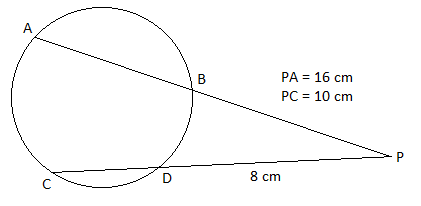PA × PB = PC × PD

16 × PB = 10 × 8

PB = 80/16

PB = 5 cm

Now,

PA = AB + PB

16 = AB + 5

AB = 16 – 5

AB = 11 cm.

Question 2

(a) The common factor of 2x2 + 5x + k and 2x2 + 3x + l is (2x – 1). Find the values of k and l. 

Solution:

Let f(x) = 2x2 + 5x + k and p(x) = 2x2 + 3x + l

Given that, (2x – 1) is the factor of f(x) and g(x).

Thus, f(½) = 0 and p(½) = 0

Now,

f(½) = 0

2(½)2 + 5(½) + k = 0

2(¼) + (5/2) + k = 0

(½) + (5/2) + k = 0

(6/2) + k = 0

3 + k = 0

k = -3

And

p(½) = 0

2(½)2 + 3(½) + l = 0

2(¼) + (3/2) + l = 0

(½) + (3/2) + l = 0

(4/2) + l = 0

2 + l = 0

l = -2

Therefore, k = -3 and l = -2.

(b)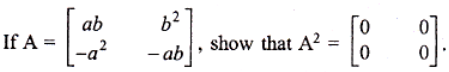Solution:

Given,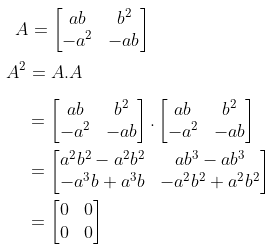(c) Solve 7 ≤ 4x + 2 ≤ 12, x ∈ R. Graph the solution set on the number line. 

Solution:

7 ≤ 4x + 2 ≤ 12, x ∈ R

7 – 2 ≤ 4x ≤ 12 – 2

5 ≤ 4x ≤ 10

(5/4) ≤ x ≤ (10/4)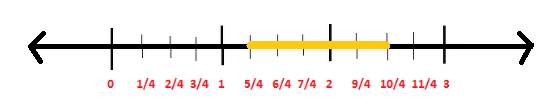Question 3

(a) The marks of 20 students in a test were as follows:

10, 15, 14, 11, 10, 8, 10, 6, 18, 19, 16, 14, 10, 3, 4, 20, 3, 10, 16, 10

Find:

(i) the mean

(ii) the median

(iii) the mode. 

Solution:

Given,

10, 15, 14, 11, 10, 8, 10, 6, 18, 19, 16, 14, 10, 3, 4, 20, 3, 10, 16, 10

Number of observations = 20

(i) Mean = Sum of observations/ Number of observations

= (10 + 15 + 14 + 11 + 10 + 8 + 10 + 6 + 18 + 19 + 16 + 14 + 10 + 3 + 4 + 20 + 3 + 10 + 16 + 10)/ 20

= 227/10

= 22.7

(ii) n = 20 (even)

Median = (½) [(n/2)th + (n/2 + 1)th observation]

= (½) [(20/2) th + (20/2 + 1)th observation]

= (½) [10th + 11th observation]

= (½) (19 + 16)

= 35/2

= 17.2

(iii) 10 has occurred the maximum number of times.

Therefore, mode = 10

(b) In the figure, part of a geometrical figure is given. Complete the figure so that the resulting figure is symmetrical about both the x-axis and the y-axis.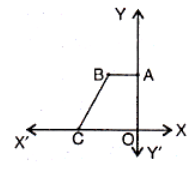Solution:

The complete figure so that the resulting figure is symmetrical about both the x-axis and the y-axis is: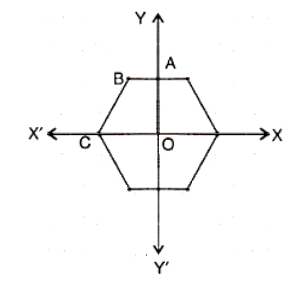(c) Show that the opposite angles of a cyclic quadrilateral are supplementary. 

Solution:

Let ABCD be a cyclic quadrilateral of a circle with centre O.

Join OB and OD.

Consider two opposite angles ∠BAD and ∠BCD.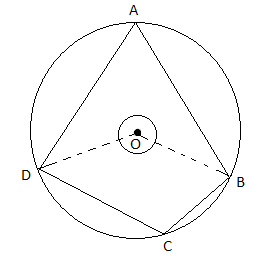We know that the angle subtended by the arc at the centre is twice the angle subtended by it at the remaining part of the circle.

Also,

reflex∠BOD = 2∠BCD….(ii)

Adding (i) and (ii),

2∠BAD + 2∠BCD = ∠BOD + reflex ∠BOD

2(∠BAD + ∠BCD) = 360°

∠BAD + ∠BCD = 360°/2

∠BAD + ∠BCD = 180°

Therefore, the opposite angles of a cyclic quadrilateral are supplementary.

Question 4

(a) Draw a circle of radius 2.5 cm. Draw two tangents to it inclined at an angle of 45° to each other. 

Solution: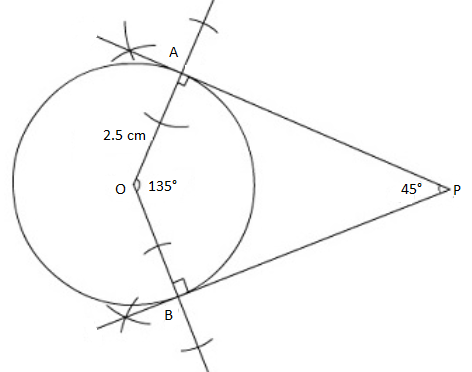Therefore, PA and PB the required tangents to the circle.

(b) Find the volume of a solid in the form of a right circular cylinder with hemispherical ends whose extreme length is 22 cm and diameter 3 cm. 

Solution:

Given,

Total length of the solid = 22 cm

Diameter of circular bases = 3 cm

Radius of cylinder = Radius of hemisphere = r = 3/2 = 1.5 cm

Height of cylinder(h) = Total length – Radius of 2 hemispheres

= 22 – 2(1.5)

= 19 cm

Volume of the solid = Volume of cylinder + 2 × Volume of hemisphere

= πr2h + 2 × (⅔)πr3

= (22/7) × 1.5 × 1.5 × 19 + (4/3) × (22/7) × 1.5 × 1.5 × 1.5

= 134.36 + 14.14

= 148.5 cm3

(c) Mr. Sagar’s savings bank account passbook entries are as follows:

 Date Particulars Withdrawn (Rs) Deposited (Rs) Balance (Rs) April 1, 2003 B/F _ _ 4175 May 5, 2003 To cheque 835 _ 3340 May 15, 2003 By clearing _ 1550 4890 July 6, 2003 To cheque 750 _ 4140 August 4, 2003 By cash _ 2300 6440 Sept. 6, 2003 To cheque 500 _ 5940

Calculate the interest on the minimum balance on or after the 10th day of the month from April to September at 4 ½ % p.a. 

Solution:

 Month Minimum balance on or after 10th day (i.e. between 10th and the last day of the month) Apr 4175 May 3340 June 4890 July 4140 Aug 6440 Sept 5940

Total = Rs. 28925

Rate of interest = R = 4 ½ % = 9/2% = 4.5%

Time = 6 months

I = PTR/100

= (28925 × 4.5 × 6)/ (12 × 100)

= 650.8125

Hence, the interest for six months is Rs. 650.8125.

Question 5

(a) Draw two intersecting lines AB and CD. Find the position of the point which is 2 cm away from AB and 1.8 cm away from CD. 

Solution:

AB and CD are two intersecting lines that intersect each other at the point O.

Construct a line EF which is parallel to AB which 2 cm away and GH which is parallel to CD (1.8 cm away) intersecting each other at point P.

Hence, P is the position of the required point.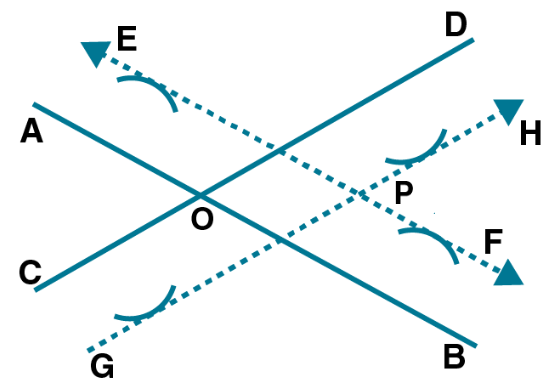(b) In how many years a sum of Rs. 6400 compounded quarterly at the rate of 5% p.a. will amount to Rs. 6561? 

Solution:

Given,

P = Rs. 6400

R = 5% p.a. = (5/4)% per quarter

Let n be the number of years.

A = Rs. 6561

A = P(1 + R/100)n

6561 = 6400 [1 + (5/400)]n

6561/ 6400 = [1 + (1/80)]n

6561/ 6400 = (81/80)n

(81/80)2 = (81/80)n

⇒ n = 2

Hence, the required number of years is 2.

(c) Angle of elevation of a cloud from a point 20 m above the surface of a lake is 30°. The angle of depression of the reflection of the cloud in the lake from the same point is 60°. Calculate the height of the cloud above the lake. 

Solution:

Let A be the surface of the lake.

C be the cloud and D be its reflection.

B be the point of observation.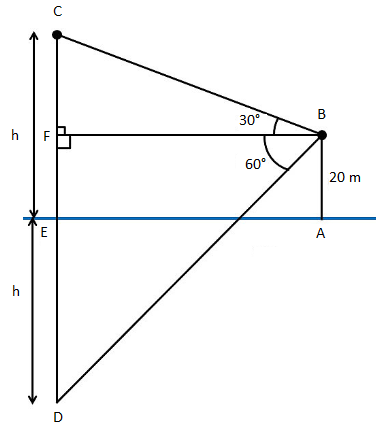AB = EF = 20 m

CE = DE = h

AE = BF

In right triangle BFC,

tan 30° = CF/BF

1/√3 = (h – 20)/ BF

BF = √3(h – 20)….(i)

In right triangle BFD,

tan 60° = DF/BF

√3 = (h + 20)/ BF

BF = (h + 20)/ √3….(ii)

From (i) and (ii),

√3(h – 20) = (h + 20)/ √3

(√3)(√3)(h – 20) = h + 20

3(h – 20) – h = 20

3h – 60 – h = 20

2h = 20 + 60

2h = 80

h = 80/2

h = 40 m

Hence, the height of the cloud above the lake is 40 m.

Question 6

(a) In the figure, AX = 2BX and CX = 2XD. Prove that:

(i) ΔAXC and ΔBXD are similar

(ii) AC || DB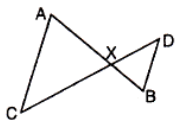Solution:

(i) Given,

AX = 2BX

CX = 2XD

∠AXC = ∠BXD (vertically opposite angles)

By SAS similarity criterion,

ΔAXC ~ ΔBXD

(ii) ∠XAC = ∠XDB (corresponding angles)

Therefore, AC || BD

(b) A manufacturer sold a dining table to a dealer for Rs. 8000. The dealer sold it to the shopkeeper at a profit of Rs. 2000. The shopkeeper sold it to the consumer at a profit of Rs. 3000. Find:

(i) the total VAT received by the government at 8%

(ii) the amount paid by the consumer inclusive of sales tax. 

Solution:

Given,

Selling price by manufacturer = Rs. 8000

Selling price by the dealer = Rs. 8000 + Rs. 2000 = Rs. 10000

Selling price by the shopkeeper = Rs. 10000 + Rs. 3000 = Rs. 13000

(i) Total VAT received by the government = (8/100) × Rs. 8000 + (8/100) × Rs. 10000 + (8/100) × Rs. 13000

= Rs. 640 + Rs. 800 + Rs. 1040

= Rs. 2480

(ii) The amount paid by the consumer inclusive of sales tax = Rs. 13000 + (8/100) × Rs. 13000

= Rs. 13000 + Rs. 1040

= Rs. 14040

(c) Two unbiased coins are tossed simultaneously. Find the probability of getting:

(iii) at least one head. 

Solution:

Given,

Two unbiased coins are tossed simultaneously.

Sample space = S = {HH, HT, TH, TT}

n(S) = 4

(i) Let A be the event of getting two heads.

A = {HH}

n(A) = 1

P(A) = n(A)/n(S) = ¼

(ii) Let B be the event of getting one head.

B = {HT, TH}

n(B) = 2

P(B) = n(B)/n(S)

= 2/4

= ½

(iii) Let C be the event of getting at least one head.

C = {HT, TH, HH}

n(C) = 3

P(C) = n(C)/ n(S)

= ¾

Question 7

(a) Construct a ΔABC in which AB = AC = 3 cm and BC = 2 cm. Using a ruler and compasses only, draw the reflection A’BC of ΔABC in BC. Draw the lines of symmetry of the figure ABA’C. 

Solution:

Given,

AB = AC = 3 cm

BC = 2 cm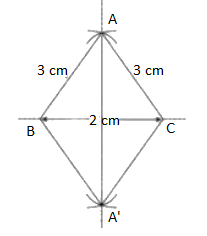AA’ and BC are the two lines of symmetry.

(b)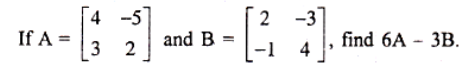Solution:

Given,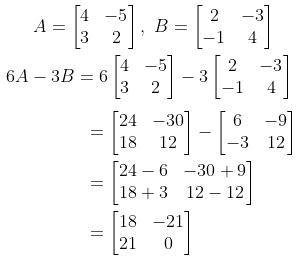(c) Using the quadratic formula, solve: [(x – 1)/ (x – 2)] + [(x – 2)/ (x – 3)] = 4. 

Solution:

[(x – 1)/ (x – 2)] + [(x – 2)/ (x – 3)] = 4

(x – 1)(x – 3) + (x – 2)(x – 2) = 4(x – 2)(x – 3)

x2 – 3x – x + 3 + x2 – 2x – 2x + 4 = 4(x2 – 3x – 2x + 6)

2x2 – 8x + 7 = 4x2 – 20x + 24

4x2 – 20x + 24 – 2x2 + 8x – 7 = 0

2x2 – 12x + 17 = 0

Comparing with the standard form ax2 + bx + c = 0,

a = 2, b = -12, c = 17

x = [-b ± √(b2 – 4ac)]/ 2a

x = [-(-12) ± √{(-12)2 – 4(2)(17)}] / 2(2)

= [12 ± √(144 – 136)]/ 4

= [12 ± √8]/ 4

= [12 ± 2√2]/ 4

= 2(6 ± √2)/ 4

= (6 ± √2)/ 2

Question 8

(a) In the figure, P is a point on AB such that AP : PB = 4 : 3 and PQ || AC. Calculate the ratio of PQ : AC.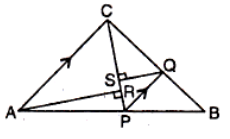Solution:

Given,

AP : PB = 4 : 3

PQ || AC

By BPT,

AP/PB = CQ/QB

CQ/QB = 4/3

⇒ BQ/BC = 3/7….(i)

Now,

∠PQB = ∠ACB (corresponding angles)

∠QPB = ∠CAB (corresponding angles)

By AA similarity,

ΔPBQ ~ ΔABC

⇒ PQ/AC = BQ/BC (by BPT)

⇒ PQ/AC = 3/7 [From (i)]

Therefore, PQ : AC = 3 : 7

(b) In what ratio does the point (-3, 7) divide the join of A(-5, 11) and B(4, -7)? 

Solution:

Let P(-3, 7) divide the line joining A(-5, 11) and B(4, -7) in the ratio λ : 1.

(-5, 11) = (x1, y1)

(4, -7) = (x2, y2)

M : n = λ : 1

Using the section formula,

P(x, y) = [(mx2 + nx1)/ (m + n), (my2 + ny1)/ (m + n)]

P(-3, 7) = [(4λ – 5)/ (λ + 1), (-7λ + 11)/ (λ + 1)]

Now,

-3 = (4λ – 5)/ (λ + 1)

-3(λ + 1) = 4λ – 5

4λ – 5 + 3λ + 3 = 0

7λ – 2 = 0

λ = 2/7

Hence, the required ratio is 2 : 7.

(c) Neha invests in 12% Rs. 25 shares of a company quoted at Rs. 36. Her income from this investment is Rs. 720. Calculate:

(i) the total amount of money invested by her in these shares

(ii) the number of shares bought by her

(iii) % return on her investment. 

Solution:

Let n be the number of shares.

Given,

Nominal value = Rs. 25

Market value = Rs. 36

Nominal value of n shares = Rs. 25n

Dividend = 12% of Rs. 25n

Income from the shares = Rs. 720

720 = (12/100) × 25n

n = (720 × 100)/ (25 × 12)

n = 240

Total investment = Market value × n

= Rs. 36 × 240

= Rs. 8640

Percentage of return on investment = (Income/ Total investment) × 100

= (720/ 8640) × 100

= 83.33%

Question 9

(a) Find the equation of a line that passes through (1, 3) and is parallel to the line y = -2x + 4. 

Solution:

The line parallel to y = -2x + 4 will have the same slope.

By comparing y = mx + c

Slope = m = -2

Given point is (1, 3)

Equation of the line passing through (x1, y1) and having slope m is:

y – y1 = m(x – x1)

y – 3 = -2(x – 1)

y – 3 = -2x + 2

y = -2x + 2 + 3

y = -2x + 5

(b) In the figure, O is the centre of the circle. If ∠PAO = 30° and ∠PBO = 40°, find:

(i) ∠APB

(ii) ∠AOB.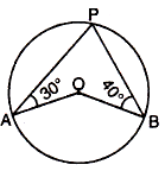Solution:

Given,

∠PAO = 30°

∠PBO = 40°

Join AB (chord) such that OA and OB are angle bisectors of ∠PAB and ∠PBA respectively.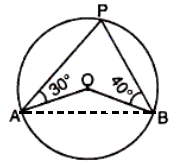Thus, ∠OAB = 30° and ∠OBA = 40°

In triangle AOB,

∠OAB + ∠OBA + ∠AOB = 180° (by the angle sum property of a triangle)

30° + 40° + ∠AOB = 180°

∠AOB = 180° – 30° – 40°

∠AOB = 110°

And

∠APB = (½) × ∠AOB

= (½) × 110°

= 55°

(c) The area of the quadrant OAB of a circle is 9 ⅝ cm2. Calculate:

(i) OA

(ii) the perimeter of the quadrant.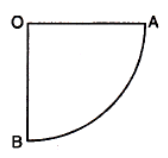Solution:

(i) Let r be the radius of the quadrant of the circle.

i.e. OA = OB = r

Given,

Area of the quadrant OAB = 9 ⅝ cm2 = 77/8 cm2

(πr2)/4 = 77/8

(22/7) × r2 = (77 × 4)/ 8

r2 = (77 × 7)/ (2 × 22)

r2 = (7 × 7)/ (2 × 2)

r2 = (7/2)2

r = 7/2 cm

Therefore, OA = 7/2 cm

(ii) Perimeter of quadrant = (¼) 2πr

= (¼) × 2 × (22/7) × (7/2)

= 11/2 cm

Question 10

(a) Find the value of m such that the lines 3xm + 3y = 5 and y = 1 – 2x are perpendicular to each other. 

Solution:

Given,

3xm + 3y = 5

3y = -3xm + 5

y = (-3mx/3) + (5/3)

y = -mx + (5/3)

Slope = m1 = m

And

y = 1 – 2x

y = -2x + 1

Slope = m2 = -2

Given that, the two lines are perpendicular to each other.

m1m2 = -1

(m)(-2) = -1

-2m = -1

m = ½

(b) Draw an Ogive for the following distribution and hence estimate the median.

 Marks 10 – 20 20 – 30 30 – 40 40 – 50 50 – 60 60 – 70 70 – 80 Frequency 6 7 9 10 8 7 3


Solution:

Less than cumulative frequency distribution table:

 Marks Cumulative frequency Less than 20 6 Less than 30 13 Less than 40 22 Less than 50 32 Less than 60 40 Less than 70 47 Less than 80 50

N = 50

N/2 = 50/2 = 25

Ogive: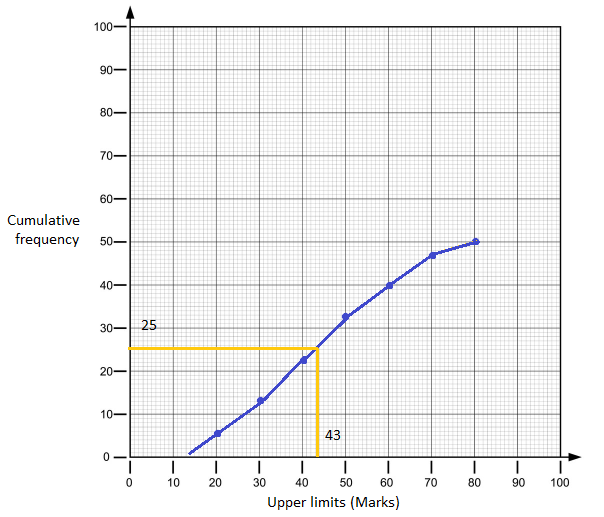Therefore, the median = 43

Question 11

(a) Show that the equation x2 + 2px – 3 = 0 has real and distinct roots for all values of p. 

Solution:

Given,

x2 + 2px – 3 = 0

Comparing with the standard form ax2 + bx + c = 0,

a = 1, b = 2p, c = -3

Condition for real and distinct roots is:

b2 – 4ac > 0

(2p)2 – 4(1)(-3) > 0

4p2 + 12 = 0

4(p2 + 3) > 0

p2 + 3 > 0

For any value of p, the above condition will be satisfied.

Thus, for any value of p, p2 + 3 > 0 is always true.

Hence, the given equation has real and distinct roots for all the values of p.

(b) Prove that: [1/ (1 – sin θ)] + [1/ (1 + sin θ)] = 2 sec2θ. 

Solution:

LHS = [1/ (1 – sin θ)] + [1/ (1 + sin θ)]

= [(1 + sin θ) + (1 – sin θ)] / [(1 + sin θ) (1 – sin θ)]

= (1 + sin θ + 1 – sin θ) / (12 – sin2θ)

= 2/ (1 – sin2θ)

Using the identity sin2A + cos2A = 1,

= 2/cos2θ

= 2 sec2θ

= RHS

Hence proved.

(c) From the following frequency distribution, find mean, mode and median.

 Variate 10 11 13 15 18 20 24 Frequency 4 3 7 1 5 2 3


Solution:

 Variate (x) Frequency (f) fx Cumulative frequency 10 4 40 4 11 3 33 7 13 7 91 14 15 1 15 15 18 5 90 20 20 2 40 22 24 3 72 25 ∑f = 25 ∑fx = 381

Mean = ∑fx/ ∑f

= 381/25

= 15.24

N = ∑f = 25

N/2 = 25/2

Cumulative frequency greater than and nearest to N/2 is 14.

Median = 13

Highest frequency = 7

Hence, the mode = 13